• 通过学习与借鉴写的第十二届蓝桥杯单片机。第一次写，不足请多多指教，喷请轻点。 主程序 #include"main.h" extern bit timer_flag; //使运行中断时与主函数不冲突 extern u8 key_flag; //判断按键是否按下 ...
通过学习与借鉴写的第十二届蓝桥杯单片机 简单易懂
全部程序请点击这里
提取码：azxy
主程序
#include"main.h"

extern bit timer_flag;				//使运行中断时与主函数不冲突
extern u8 key_flag;					//判断按键是否按下
//0:按下
//1：没按下
extern u8 key_num;					//按键反馈值
extern u8 smg_change;			//8个数码管显示
extern u8 code duan;			//0~9和要显示的数值

u8 mod_led=0xff;					//定义LED灯开关状态
u16 temp;							//温度值
u16 wdcs=25;						//在参数温度时，温度参数变量
u16 wdcs_now=25;					//在程序运行时，生效温度参数
u16 dac=0;							//待输出的ADC电压值
u16 stan=0;							//界面切换
//0：为温度界面
//1：为参数设置界面
//2：DAC输出界面

u16 f_mod=0;					    //模式切换
//0：模式1
//1：模式2

/*==========================普通延迟函数============================*/
void delay(u16 i)
{
while(i--);
}

/*==========================锁存器选择函数 =========================*/
void init_74hc573(num)
{
switch(num)
{
case 4: P2=(P2&0x1f)|0x80; break;	  //选择LED控制
case 5: P2=(P2&0x1f)|0xa0; break;	  //选择蜂鸣器和继电器控制
case 6: P2=(P2&0x1f)|0xc0; break;	  //选择数码管位选
case 7: P2=(P2&0x1f)|0xe0; break;	  //选择数码管段选
case 0: P2=(P2&0x1f)|0x00; break;	  //所有不选择
}
}

/*==========================系统初始化============================*/
void init_system()
{
init_74hc573(4);				//关闭LED灯
P0=0xff;
init_74hc573(5);			    //关闭蜂鸣器
P0=0x00;
}

/*========================LED输出控制函数==========================*/
void set_led(u8 aa)
{
init_74hc573(4);
P0=aa;
init_74hc573(0);
}

/*=======================数码管输出控制函数=========================*/
void set_smg(u8 bb)
{
init_74hc573(7);
P0=bb;
init_74hc573(6);
P0=0x00;
}

/*=======================温度显示界面函数==========================*/
void smg_wd()
{
temp=read_18b20float();
set_led(0xff);
mod_led=(mod_led|0xfe)&0xfd;
set_led(mod_led);

set_smg(0xff);
smg_change=duan;
smg_change=duan;
smg_change=duan;
smg_change=duan;
smg_change=duan[temp / 1000];
smg_change=duan[temp % 1000 / 100]+0x80;
smg_change=duan[temp % 100 / 10];
smg_change=duan[temp % 10];
set_smg(0xff);
}

/*=======================参数设置界面函数==========================*/
void smg_cs()
{
set_led(0xff);
mod_led=(mod_led|0xfe)&0xfb;
set_led(mod_led);

set_smg(0xff);
smg_change=duan;
smg_change=duan;
smg_change=duan;
smg_change=duan;
smg_change=duan;
smg_change=duan;
smg_change=duan[wdcs/ 10];
smg_change=duan[wdcs% 10];
set_smg(0xff);
}

/*=======================DAC输出界面函数==========================*/
void smg_dac()
{
set_led(0xff);
mod_led=(mod_led|0xfe)&0xf7;
set_led(mod_led);

set_smg(0xff);
smg_change=duan;
smg_change=duan;
smg_change=duan;
smg_change=duan;
smg_change=duan;
smg_change=duan[dac/100]+0x80;
smg_change=duan[(dac/10)%10];
smg_change=duan[dac% 10];
set_smg(0xff);
}

/*=======================运行界面函数==========================*/
void running()
{
u8 dat_dac;
u16 tmp=0;
temp=read_18b20float();
switch(f_mod)
{
case 0:
if(temp<(wdcs_now*100))
{
dac=0;
}
else
{
dac=500;
}
break;
case 1:
if(temp<2000)
{
dac=100;
}
else if(temp<4000)
{
tmp=(temp-2000)*15;
dac=(tmp/100)+100;
}
else
{
dac=400;

}
break;
}
dat_dac=dac*0.51;
pcf8591(dat_dac);
}

/*=======================显示界面函数==========================*/
void displaysmg()
{
switch(f_mod)
{
case 0:
mod_led=0xfe;
break;
case 1:
mod_led=0xff;
break;
}
switch(stan)
{
case 0:
running();
smg_wd();
break;
case 1:
running();
smg_cs();
break;
case 2:
running();
smg_dac();
break;

}
}

/*=======================矩阵按键函数==========================*/
void key_cin()
{
if(key_num==8)						 //如果S5按下
{
f_mod++;
if(f_mod==1) stan=0;
if(f_mod==2)
{
f_mod=0;
stan=0;
}
while(key_flag==0)				   //按键释放
{
displaysmg();
delay(1000);
}
}
if(key_num==12)					  	  //如果S4按下
{
stan++;
if(stan==1)
{
wdcs=wdcs_now;
}
else if(stan==2)
{
wdcs_now=wdcs;

}
else if(stan==3) stan=0;
while(key_flag==0)				 //按键释放
{
displaysmg();
delay(1000);
}

}
if(key_num==9)						//如果S9按下
{
if(stan==1)
{
wdcs++;
}
while(key_flag==0)				//按键释放
{
displaysmg();
delay(1000);
}
}
if(key_num==13)					   //如果S8按下
{
if(stan==1)
{
wdcs--;
}
while(key_flag==0)			  //按键释放
{
displaysmg();
delay(1000);
}
}
}

/*=======================主函数==========================*/
void main()
{
init_system();			   //系统初始化
Timer0Init();			   //中断
while(1)
{
if(key_flag==1)		  //按键消抖
{
key_sd();
key_flag=0;
}
running();
displaysmg();
key_cin();
displaysmg();
}
}



展开全文单片机
•java
• 2021年第十二届蓝桥杯省赛B组第一场（真题+全部题解）

暴力杯？dp杯！
重铸国二荣光，省三义不容辞

感谢评论区的大佬给出的思路还有指正的一些错误地方，现都已更正，当然如果还有其他更优解法的也欢迎评论区指出
c/c++和Java的B组题目都已更新，正在备考的同学欢迎收藏，题解主要用的是Java语言，最后两题都是引用y总(yxc)的题解，我太菜了

文章目录试题A: 空间(c/c++)试题A: ASCII码(Java)试题B: 卡片试题C: 直线试题 D: 货物摆放试题 E: 路径试题 F: 时间显示试题 G: 砝码称重(c/c++)试题G: 最少砝码(Java)试题 H: 杨辉三角形试题 I: 双向排序试题 J: 括号序列
试题A: 空间(c/c++)

小蓝准备用 256MB 的内存空间开一个数组，数组的每个元素都是 32 位二进制整数，如果不考虑程序占用的空间和维护内存需要的辅助空间，请问256MB 的空间可以存储多少个 32 位二进制整数？

#include <iostream>
#include <algorithm>
using namespace std;
int main()
{
cout<<256*1024*1024/4;
return 0;
}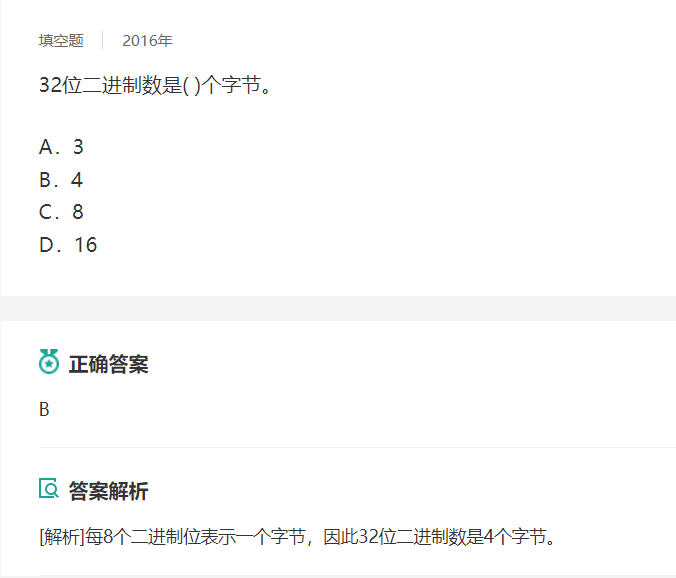ans = 67108864
试题A: ASCII码(Java)

大致题意是已知‘A’的ascii码，求‘L’的ascii码

public class Main{
public static void main(Stirng args[]){
System.out.println((int)'L');
}
}

ans = 76
试题B: 卡片

小蓝有很多数字卡片，每张卡片上都是数字 0 到 9。
小蓝准备用这些卡片来拼一些数，他想从 1 开始拼出正整数，每拼一个，就保存起来，卡片就不能用来拼其它数了。
小蓝想知道自己能从 1 拼到多少。
例如，当小蓝有 30 张卡片，其中 0 到 9 各 3 张，则小蓝可以拼出 1 到 10，但是拼 11 时卡片 1 已经只有一张了，不够拼出 11。
现在小蓝手里有 0 到 9 的卡片各 2021 张，共 20210 张，请问小蓝可以从 1拼到多少？
提示：建议使用计算机编程解决问题。

import java.util.*;
public class Main{

static Map<Integer,Integer>map;
public static void main(String[] args) {
map = new HashMap<Integer, Integer>();
for(int i=0;i<=9;i++) {
map.put(i,2021);
}
for(int i=1;i<100000;i++) {
if(pd(i))System.out.println(i);
else break;
}
}
public static boolean pd(int idx) {
int index = idx;
Integer a;
while(index>0) {
a = map.get(index%10);
if(a==0)return false;
map.put(index%10, a-1);
index/=10;
}
return true;
}
}



Console:
1
2
3
…………
3177
3178
3179
3180
3181

ans = 3181
试题C: 直线

在平面直角坐标系中，两点可以确定一条直线。如果有多点在一条直线上，  那么这些点中任意两点确定的直线是同一条。
给定平面上 2 × 3 个整点 {(x, y)|0 ≤ x < 2, 0 ≤ y < 3, x ∈ Z, y ∈ Z}，即横坐标是 0 到 1 (包含 0 和 1) 之间的整数、纵坐标是 0 到 2 (包含 0 和 2) 之间的整数的点。这些点一共确定了 11 条不同的直线。
给定平面上 20 × 21 个整点 {(x, y)|0 ≤ x < 20, 0 ≤ y < 21, x ∈ Z, y ∈ Z}，即横坐标是 0 到 19 (包含 0 和 19) 之间的整数、纵坐标是 0 到 20 (包含 0 和 20) 之间的整数的点。请问这些点一共确定了多少条不同的直线。

//暴力即可，但要进行特判，如果两个点连成的线段不是所在的直线中该规定坐标系内最长的线段则不记数
public class Main {

static Node[]list;
static int ans;
public static void main(String[] args) {
solve(2,3);
solve(3,3);
solve(20,21);
}
public static void solve(int m,int n) {//m*n的平面坐标系
ans=0;
list = new Node[m*n];
int cur=0;
for(int x=0;x<m;x++) {
for(int y=0;y<n;y++) {
list[cur++] = new Node(x,y);
}
}

int xfar,yfar,gcd;
for(int i=0;i<m*n-1;i++) {
for(int j=i+1;j<m*n;j++) {
xfar = list[j].x-list[i].x;
yfar = list[j].y-list[i].y;
if(xfar==0 || yfar==0)continue;//垂直和水平的线先排除掉，不然求公约数有点麻烦
gcd = gcd(xfar,yfar);
xfar/=gcd;
yfar/=gcd;//现在xfar和yfar代表的是两点之间下x，y距离的最小整数比
//如果这根线的左端点往左移动一个最小距离还是在图形的范围内的话就排除
if(list[i].x-xfar>=0 && list[i].y-yfar>=0 && list[i].y-yfar<n)continue;
//如果这根线的右端点往右移动一个最小距离还是在图形的范围内的话就排除
if(list[j].x+xfar<m && list[j].y+yfar>=0 && list[j].y+yfar<n)continue;
//这样保证每根线只有图形中最长的那根会被计算进去，中间短的线会被continue

//				System.out.println((i+1)+" "+(j+1)+" "+xfar+" "+yfar);
//				System.out.println(list[i]+" "+list[j]);
//				System.out.println("------------------");
ans++;
}
}
ans+=(m+n);
System.out.println(ans);
}
public static int gcd(int a,int b){//求最大公约数
int mid=a;
a = Math.abs(a);
b = Math.abs(b);
if(b>a) {a=b;b=mid;}
if(b == 0)return a;
return gcd(b,a%b);
}

}
class Node{
int x;
int y;
public Node(int x,int y) {
// TODO Auto-generated constructor stub
this.x = x;
this.y = y;
}
@Override
public String toString() {
// TODO Auto-generated method stub
return x+" "+y;
}
}



控制台输出的数据：
11
20
40257
以第二组数据为例：3，3，把中间注解的部分取消在控制台可以得到：

1 6 1 2      1表示起始点，6表示终止点，1表示xfar，2表示yfar
0 0 1 2      0，0是起始点的坐标，1，2是终止点的坐标
------------------
1 8 2 1
0 0 2 1
------------------
1 9 1 1
0 0 2 2
------------------
2 4 1 -1
0 1 1 0
------------------
2 6 1 1
0 1 1 2
------------------
2 7 2 -1
0 1 2 0
------------------
2 9 2 1
0 1 2 2
------------------
3 4 1 -2
0 2 1 0
------------------
3 7 1 -1
0 2 2 0
------------------
3 8 2 -1
0 2 2 1
------------------
4 8 1 1
1 0 2 1
------------------
4 9 1 2
1 0 2 2
------------------
6 7 1 -2
1 2 2 0
------------------
6 8 1 -1
1 2 2 1
------------------
20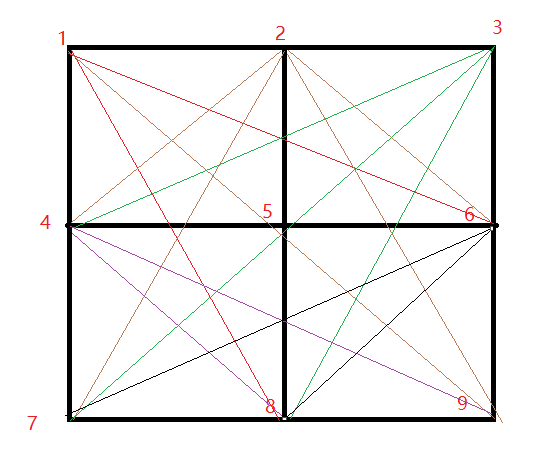16、18、19、24、26、27、29、34、37、38、48、49、67、68
再加上垂直的三条和水平的三条一共20条，其中15，57等线段因为不是所属直线上在该图形内的最长的线段所以没被记录。

最后答案为：
ans = 40257

补充：
Java组的同学也可以用set来去重，我这里用的HashMap，其实是一样的，不过要注意的是得重写斜率和截距所在类的hashCode和equals方法

import java.util.*;

public class lan {

static Node[]list;
static int ans;
public static void main(String[] args) {
setSolve(20,21);
}

public static void setSolve(int m,int n) {
ans=0;
list = new Node[m*n];
Map<Line,Integer>map = new HashMap();
Integer a;

int cur=0;
for(double x=0;x<m;x++) {
for(double y=0;y<n;y++) {
list[cur++] = new Node(x,y);
}
}
for(int i=0;i<m*n;i++) {
for(int j=i+1;j<m*n;j++) {
if(list[i].x==list[j].x || list[j].y==list[i].y)continue;
//这里一大堆其实就是求斜率和截距，用自己喜欢的方式写就行，我懒得分开了
a=map.get(new Line((list[j].y-list[i].y)/(list[j].x-list[i].x),(list[j].x*list[i].y-list[j].y*list[i].x)/(list[j].x-list[i].x)));
if(a==null) {
ans++;
map.put(new Line((list[j].y-list[i].y)/(list[j].x-list[i].x),(list[j].x*list[i].y-list[j].y*list[i].x)/(list[j].x-list[i].x)),1);
}
}
}
System.out.println(map.size()+m+n);
}
}
class Node{
//这里和上个解法不同的是要注意用double，因为待会要进行截距和斜率的计算
public double x;
public double y;
public Node(double x,double y) {
// TODO Auto-generated constructor stub
this.x = x;
this.y = y;
}
}

class Line{
public Double k;
public Double b;
public Line(Double k,Double b) {
// TODO Auto-generated constructor stub
this.k = k;
this.b = b;
}

@Override
public boolean equals(Object obj) {
// TODO Auto-generated method stub
return (this.k.equals(((Line)obj).k) && ((Line)obj).b.equals(this.b));
}

@Override
public int hashCode() {
// TODO Auto-generated method stub
return k.hashCode()+b.hashCode();
}
}



至于为什么要重写equals和hashCode也是常考的面试题，有时间可以再写个博客讲讲

最后答案也是  40257
试题 D: 货物摆放

小蓝有一个超大的仓库，可以摆放很多货物。
现在，小蓝有 n 箱货物要摆放在仓库，每箱货物都是规则的正方体。小蓝规定了长、宽、高三个互相垂直的方向，每箱货物的边都必须严格平行于长、宽、高。
小蓝希望所有的货物最终摆成一个大的立方体。即在长、宽、高的方向上分别堆 L、W、H 的货物，满足 n = L × W × H。
给定 n，请问有多少种堆放货物的方案满足要求。
例如，当 n = 4 时，有以下 6 种方案：1×1×4、1×2×2、1×4×1、2×1×2、2 × 2 × 1、4 × 1 × 1。
请问，当 n = 2021041820210418 （注意有 16 位数字）时，总共有多少种方案？
提示：建议使用计算机编程解决问题。

#python是世界上最好的语言
import math
n = 2021041820210418
ans=0
ls = []
for i in range(1,int(math.sqrt(n))):
if n%i==0:
ls.append(int(i))
ls.append(int(n/i))
size=len(ls)
for i in range(size):
for j in range(size):
for p in range(size):
if ls[i]*ls[j]*ls[p]==n:
ans=ans+1
print(ans)填空题，不用管时间，直接暴力把所有因数求出来然后三重循环
试题 E: 路径

小蓝学习了最短路径之后特别高兴，他定义了一个特别的图，希望找到图中的最短路径。
小蓝的图由 2021 个结点组成，依次编号 1 至 2021。
对于两个不同的结点 a, b，如果 a 和 b 的差的绝对值大于 21，则两个结点之间没有边相连；如果 a 和 b 的差的绝对值小于等于 21，则两个点之间有一条长度为 a 和 b 的最小公倍数的无向边相连。
例如：结点 1 和结点 23 之间没有边相连；结点 3 和结点 24 之间有一条无向边，长度为 24；结点 15 和结点 25 之间有一条无向边，长度为 75。
请计算，结点 1 和结点 2021 之间的最短路径长度是多少。
提示：建议使用计算机编程解决问题。

用辗转相除法求最大公约数 x%y ? gcd(y, x % y) : y
然后用最大公约数求最小公倍数 x * y / gcd(x, y)
这样就可以得到一个2021*2021的无向图，用邻接矩阵存储这张图，然后dijkstra算法求最短路径
关于dijkstra的内容不熟悉的可以看这篇博文
dijkstra算法求最短路径
import java.text.DecimalFormat;
import java.util.Scanner;

public class Main {

public static void main(String[] args) {
int map[][] = new int;//存大点下面初始化的时候不用考虑数据越界

for(int i=1;i<=2021;i++) {
for(int j=i;j<=i+21;j++) {
//大的数放前面，或者在求最大公约数的时候加一个交换操作
map[i][j] = lcm(j,i);
}
}
boolean bj[] = new boolean;//用来表示该点是否已经是最短
int dis[] = new int;//用来存储源点到其他顶点的初始路径
for(int i=1;i<=2021;i++)dis[i]=map[i];

int min,minIdx=0;
while(!bj) {//如果2021点的最短路径还没求到就一直循环
min = Integer.MAX_VALUE;
for(int i=2;i<=2021;i++) {
if(!bj[i] && dis[i]!=0 && dis[i]<min) {
min = dis[i];
minIdx = i;
}
}
bj[minIdx]=true;
for(int i=minIdx+1;i<=minIdx+21;i++) {
if(map[minIdx][i]!=0) {//我初始化的时候没有把邻接矩阵的初始值赋一个大的数，所以这里要特判一下
if(dis[i]==0)dis[i] = dis[minIdx]+map[minIdx][i];
else{
if(dis[minIdx]+map[minIdx][i]<dis[i])dis[i]=dis[minIdx]+map[minIdx][i];
}
}
}
}
System.out.println(dis);

}
public static int gcd(int x,int y) {//欧几里得算法
return x%y!=0 ? gcd(y, x % y) : y;
}

public static int lcm(int x,int y) {//最小公倍数
return x * y / gcd(x, y);
}
}


试题 F: 时间显示

小蓝要和朋友合作开发一个时间显示的网站。在服务器上，朋友已经获取了当前的时间，用一个整数表示，值为从 1970 年 1 月 1 日 00:00:00 到当前时刻经过的毫秒数。
现在，小蓝要在客户端显示出这个时间。小蓝不用显示出年月日，只需要显示出时分秒即可，毫秒也不用显示，直接舍去即可。
给定一个用整数表示的时间，请将这个时间对应的时分秒输出。

【输入格式】
输入一行包含一个整数，表示时间。
【输出格式】
输出时分秒表示的当前时间，格式形如 HH:MM:SS，其中 HH 表示时，值
为 0 到 23，MM 表示分，值为 0 到 59，SS 表示秒，值为 0 到 59。时、分、秒
不足两位时补前导 0。
【样例输入 1】
46800999
【样例输出 1】
13:00:00
【样例输入 2】
1618708103123
【样例输出 2】
01:08:23
【评测用例规模与约定】
对于所有评测用例，给定的时间为不超过 10^18 的正整数。

import java.text.DecimalFormat;
import java.util.Scanner;

public class Main{

public static void main(String[] args) {
Scanner sc = new Scanner(System.in);
DecimalFormat df = new DecimalFormat("00");
long time = sc.nextLong()/1000;
int hour,minute,cecond;

time %= 3600*24;
hour = (int)time/3600;
time %= 3600;
minute = (int)time/60;
cecond = (int)time%60;

System.out.println(df.format(hour)+":"+df.format(minute)+":"+df.format(cecond));
}

}


试题 G: 砝码称重(c/c++)

你有一架天平和 N 个砝码，这 N 个砝码重量依次是 W1, W2, · · · , WN。请你计算一共可以称出多少种不同的重量？
注意砝码可以放在天平两边。

【输入格式】
输入的第一行包含一个整数 N。
第二行包含 N 个整数：W1, W2, W3, · · · , WN。
【输出格式】
输出一个整数代表答案。
【样例输入】
3
1 4 6
【样例输出】
10
【样例说明】
能称出的 10 种重量是：1、2、3、4、5、6、7、9、10、11。
1 = 1；
2 = 6 − 4 (天平一边放 6，另一边放 4)；
3 = 4 − 1；
4 = 4；
5 = 6 − 1；
6 = 6；
7 = 1 + 6；
9 = 4 + 6 − 1；
10 = 4 + 6；
11 = 1 + 4 + 6。
【评测用例规模与约定】
对于 50% 的评测用例，1 ≤ N ≤ 15。
对于所有评测用例，1 ≤ N ≤ 100，N 个砝码总重不超过 100000。

原题题解：长沙理工大学第十二届ACM大赛 D题

物品可以放左边也可以放在右边
其实可以看成一个物品可以作为重量 $ai$
也可以作为$-ai$
那么看成$2n$个物品来一次$01$背包即可
但是这里稍微有点变化,物品的价值可能是负数
那么如果负数也按照我们倒序枚举会有问题,负数应该正序枚举
负数正序枚举能保证状态没有重复使用一个物品
当然如果你用的是二维数组来$dp$就不需要分两次了

#include <bits/stdc++.h>
using namespace std;
const int N = 1e4 + 10;
int dp[N], a[N], n, m, sum;
int main() {
while (scanf("%d", &n) != EOF) {
memset(dp, 0, sizeof dp);
sum = 0;
for (int i = 1; i <= n; i++) {
scanf("%d", &a[i]);
sum += a[i];
}
dp = 1;
for (int i = 1; i <= n; i++) {//01背包，如何直接凑得我们想要的值。
for (int j = sum; j >= a[i]; j--) {
dp[j] |= dp[j - a[i]];
}
}
for (int i = 1; i <= n; i++) {
//01背包, 从后往前做一个01背包删除a[i]，通过得到一个差值，来凑得我们想要的值。
for (int j = 1; j <= sum - a[i]; j++) {
dp[j] |= dp[j + a[i]];
}
}
scanf("%d", &m);
for (int i = 1; i <= m; i++) {
int x;
scanf("%d", &x);
puts(x <= sum && dp[x] ? "YES" : "NO");
}
}
return 0;
}

来自牛客id：issue是云哥的小迷×呀

这道题统计数量的话直接遍历dp[i]记录有多少true就行了

试题G: 最少砝码(Java)

你有一架天平。现在你要设计一套砝码，使得利用这些砝码可以称出任意小于等于N的正整数重量。
那么这套砝码最少需要包含多少个砝码？
注意砝码可以放在天平两边。
【输入格式】
输入一个整数 N。
【输出格式】
输出一个整数代表答案。
【样例输入】
7
【样例输出】
3
【样例说明】
3个砝码重量是1、4、6，可以称出1至7的所有重量。
1 = 1；
2 = 6 - 4（天平一边放6，另一边放4）；
3 = 4 - 1；
4 = 4；
5 = 6 - 1；
6 = 6；
7 = 1 + 6；
少于3个砝码不可能称出1至7的所有重量。
【评测用例规模与约定】
对于所有评测用例，1 ≤ N ≤ 1000000000。

当砝码为{1}的时候，可以称出任意小于等于1的正整数重量。
当砝码为{1,3}的时候，可以称出任意小于等于4的正整数重量。
当砝码为{1,3,9}的时候，可以称出任意小于等于13的正整数重量。
当砝码为{1,3,9,27}的时候，可以称出任意小于等于40的正整数重量。
当砝码为{1,3,9,27,81}的时候，可以称出任意小于等于121的正整数重量。

思路：如果前$n$个数可以凑成任意小于等于$m$的正整数重量,那么第$n+1$个数设为$y$，$y$只需要满足$y-m=m+1$，这时$y$的值就是在题意中所表示的最大值,证明：

前$m$个正整数可以由前$n$个砝码称出来的
可以想象成第$n+1$个的砝码放在天平右边，天平左边依次放$m$到1重量的砝码，则这个阶段可以称出的范围是[$y-m$,$y-1$]
天平右边放第$n+1$个砝码，左边只放要称的物品，这时能被称出的物品重量为$y$
天平右边放第$n+1$个砝码加上重量由1到$m$的砝码，左边放物品，则这个阶段可以称出的范围是[$y+1$,$y+m$]

合在一起用这种称量方法，第$n+1$个砝码可以称出的范围是[1,m] & [$y-m$,$y+m$],当$y-m$ = $m+1$的时候，第$n+1$个砝码所能称量的范围最大。

由此可以得到规律：当砝码为$x$个的时候，最多可以称出任意小于等于$3^0+3^1+3^2+……+3^{x-1}$的正整数重量

import java.util.*;
public class Main {

public static void main(String[] args) {
Scanner sc = new Scanner(System.in);
long n = sc.nextLong();
long ans = 0;
while(n>0) {
n -= Math.pow(3, ans);
ans++;
}
System.out.println(ans);
}
}

试题 H: 杨辉三角形

下面的图形是著名的杨辉三角形：
如果我们按从上到下、从左到右的顺序把所有数排成一列，可以得到如下数列：
1, 1, 1, 1, 2, 1, 1, 3, 3, 1, 1, 4, 6, 4, 1, …
给定一个正整数 N，请你输出数列中第一次出现 N 是在第几个数？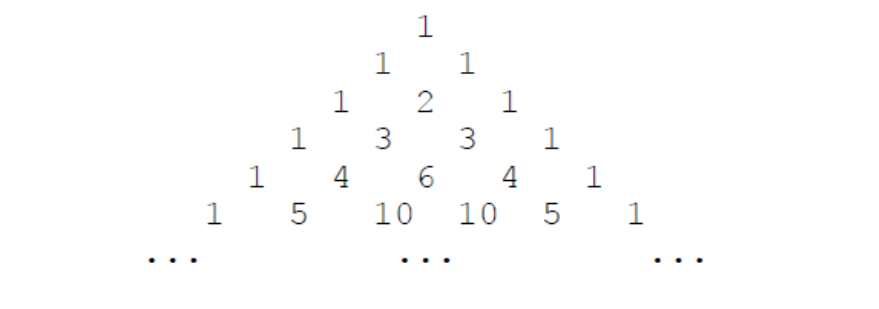【输入格式】
输入一个整数 N。
【输出格式】
输出一个整数代表答案。
【样例输入】
6
【样例输出】
13
【评测用例规模与约定】
对于 20% 的评测用例，1 ≤ N ≤ 10；
对于所有评测用例，1 ≤ N ≤ 1000000000。

该题需要掌握的前置知识：

组合数的性质 ：$C_m^n$ = $C_{m-1}^{n-1}$ + $C_{m-1}^n$
杨辉三角的性质：$每个数等于它上方两数之和。$

故有：

杨辉三角的第${m-1}$行的第$n-1$个数的值就等于$C_m^n$
杨辉三角每行正序的第i个数和逆序的第i个数的值相等
杨辉三角第 $i$ 行的前 $(i+1)/2$ 个数递增
杨辉三角第 $j$ 列从上往下的值依次递增

因此在本题中：

只需要考虑每行前一半的数即可
如果 $n$ < $C_m^n$ 则不存在 { $n$ $\geq$ $C_i^j$    |  ${i>m}$  ,  $j\geq n$}, 在$i$>$m$的情况下，$n$只可能出现在{$C_i^j$ | $i$ $>$m, $j$ $<$n   }里面
按照上面这个性质，那我们肯定能找到非常优化的解决方案，但稍微暴力一点，我们只要找到$C_m^2$的值大于$10^{10}$,且还没找到对应的n，则n第一次出现的地方一定是$C_n^1$,也就是杨辉三角的第n+1行的第2个数

如果同学们在考场中可以按win+r输入calc，按下回车然后计算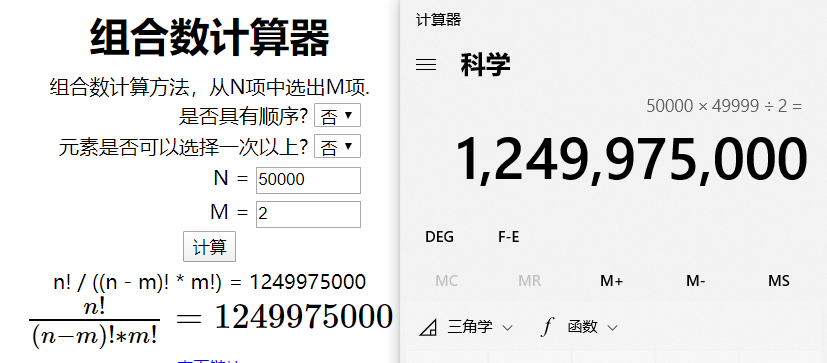可以得到大概五万行后的第二个数字就比$10^{10}$大了

import java.util.Scanner;

public class Main{

public static void main(String[] args) {
Scanner sc = new Scanner(System.in);
long n;
while(sc.hasNext()) {
System.out.println(solve(sc.nextLong()));
}
}
public static long solve(long n) {
long res = 0l;
long san[] = new long;
san=1;
//san[x]表示当前层的第x个位置，这是为了方便后面在每层第一个位置执行san[j] = san[j-1]+san[j]不出现数组越界的情况
if(n==1)return 1;
long cur=0l;
for(int i=2;i<50000;i++) {
//cur现在所在的位置是上上层的最后一个位置
//然后通过+i是到了本层第一个位置，再加i-1就到了这层最后一个位置
//加一起也就是cur+2*i-2
cur+=2*i-1;
for(int j=i;j>=1;j--) {
san[j] = san[j-1]+san[j];
if(san[j]==n)res=cur;
cur--;
}
//这里一直减到了本层第一后位置后还要--，所以一层循环完cur的位置就到了上一层的最后一个位置
//下一次循环的时候i++了，所以下一次循环开始前cur的位置是上上层的最后一个位置
if(res!=0)return res;
}
//在前五万行都找不到n这个数，也就是说n这个数不可能出现在五万行以后的第二个位置
//因为五万行以后的第二个位置的值都比1000000000大，所以n第一次出现的位置必然是第n+1行的第三个位置，也就是C上标1，下标n的值

return (n+1)*n/2+2;
//第一行是1个数，第n行是n个数，而且是等差数列，用等差数列求和公式算出前n行的值加2就是n+1行第二个数的位置了
}

}


再附一个y总直播时候讲的思路，根据杨辉三角的特性二分就能快速求到答案

#include <iostream>
#include <cstring>
#include <algorithm>

using namespace std;

typedef long long LL;

int n;

LL C(int a, int b)
{
LL res = 1;
for (int i = a, j = 1; j <= b; i --, j ++ )
{
res = res * i / j;
if (res > n) return res;
}
return res;
}

bool check(int k)
{
LL l = k * 2, r = max((LL)n, l);
while (l < r)
{
LL mid = l + r >> 1;
if (C(mid, k) >= n) r = mid;
else l = mid + 1;
}
if (C(r, k) != n) return false;

cout << r * (r + 1) / 2 + k + 1 << endl;

return true;
}

int main()
{
cin >> n;
for (int k = 16; ; k -- )
if (check(k))
break;
return 0;
}

作者：yxc
链接：https://www.acwing.com/activity/content/code/content/1175304/
来源：AcWing
著作权归作者所有。商业转载请联系作者获得授权，非商业转载请注明出处。

试题 I: 双向排序

给定序列 (a1, a2, · · · , an) = (1, 2, · · · , n)，即 ai = i。
小蓝将对这个序列进行 m 次操作，每次可能是将 a1, a2, · · · , aqi 降序排列，或者将 aqi, aqi+1, · · · , an 升序排列。
请求出操作完成后的序列。

【输入格式】
输入的第一行包含两个整数 n, m，分别表示序列的长度和操作次数。接下来 m 行描述对序列的操作，其中第 i 行包含两个整数 pi
, qi 表示操作类型和参数。当 pi = 0 时，表示将 a1, a2, · · · , aqi 降序排列；当 pi = 1 时，表示
将 aqi, aqi+1, · · · , an 升序排列。
【输出格式】
输出一行，包含 n 个整数，相邻的整数之间使用一个空格分隔，表示操作
完成后的序列。
【样例输入】
3 3
0 3
1 2
0 2
【样例输出】
3 1 2
【样例说明】
原数列为 (1, 2, 3)。
第 1 步后为 (3, 2, 1)。
第 2 步后为 (3, 1, 2)。
第 3 步后为 (3, 1, 2)。与第 2 步操作后相同，因为前两个数已经是降序了。
【评测用例规模与约定】
对于 30% 的评测用例，n, m ≤ 1000；
对于 60% 的评测用例，n, m ≤ 5000；
对于所有评测用例，1 ≤ n, m ≤ 100000，0 ≤ ai ≤ 1，1 ≤ bi ≤ n。

y总题解    y总yyds

试题 J: 括号序列

给定一个括号序列，要求尽可能少地添加若干括号使得括号序列变得合法，当添加完成后，会产生不同的添加结果，请问有多少种本质不同的添加结果。两个结果是本质不同的是指存在某个位置一个结果是左括号，而另一个是右括
号。
例如，对于括号序列 ((()，只需要添加两个括号就能让其合法，有以下几种不同的添加结果：()()()、()(())、(())()、(()()) 和 ((()))。

【输入格式】
输入一行包含一个字符串 s，表示给定的括号序列，序列中只有左括号和
右括号。
【输出格式】
输出一个整数表示答案，答案可能很大，请输出答案除以 1000000007 (即
10^9 + 7) 的余数。
【样例输入】
((()
【样例输出】
5
【评测用例规模与约定】
对于 40% 的评测用例，|s| ≤ 200。
对于所有评测用例，1 ≤ |s| ≤ 5000。

y总题解


展开全文java 算法
• 第十二届蓝桥杯嵌入式第一场省赛题目，程序题+客观
• 第十二届蓝桥杯大赛软件赛省赛第二场_JC.pdf
• 第十二届蓝桥杯大赛软件赛省赛第二场_CA.pdf
• 第十二届蓝桥杯大赛软件赛省赛第二场_CB.pdf
• 蓝桥杯嵌入式——第十二届蓝桥杯嵌入式第一场省赛 目录蓝桥杯嵌入式——第十二届蓝桥杯嵌入式第一场省赛一、赛题分析二、问题总结三、代码（完整代码，功能全部实现，附有注释） 一、赛题分析 这是刚刚过去的第十二...
蓝桥杯嵌入式——第十二届蓝桥杯嵌入式第一场省赛
目录蓝桥杯嵌入式——第十二届蓝桥杯嵌入式第一场省赛一、赛题分析二、问题总结三、代码（完整代码，功能全部实现，附有注释）
一、赛题分析
这是刚刚过去的第十二届第一场嵌入式的省赛，也是我刚刚参加的。由于我用的是旧版，我们那个考场只测试了新版的环境，而旧版的软件环境有问题，和新版的存在冲突，耽搁了很久的时间，有点搞人心态。但是最终还是基本上把赛题的功能全部实现完了的，仅仅串口有一个小bug，就是第8辆车进去过后不能出来，其他的都没有什么问题，比赛的时候时间也做的比较久了，实在是不想再调试了，这个bug就没有管了，最后还有点担心进不了国赛，但是成绩下来那一刻，进国赛了，更加没想到还是全省前三，还是不错了。
比赛的时候，拿到赛题，就发现串口部分不是那么简单。所以，就先一步一步的把其他的模块先实现了，除了串口，其他的模块都很简单，非常中规中矩。接下来就搞串口部分，串口其实说难也不是很难，主要是比较麻烦。
我觉得在我们拿到赛题的时候，应该分模块来实现，首先看看用到了哪些模块， 再去看每一个模块，赛题的要求是什么。比如说输出PWM， 题目要求就是PA7输出2kHz,占空比20%，，只用到了一个通道，并且占空比和频率是固定的，所以直接就想到用定时器的PWM模式就行了，就不需要再去用什么OCTOGGLE模式浪费时间了。
我个人觉得，进国赛是比较简单的，只要选择题不要错的太多，就拿这一届来说，串口部分比较难，所以其他部分必须全部实现了，串口实现一部分，不要有太大的逻辑错误， 影响系统运行，应该进国赛都稳了吧。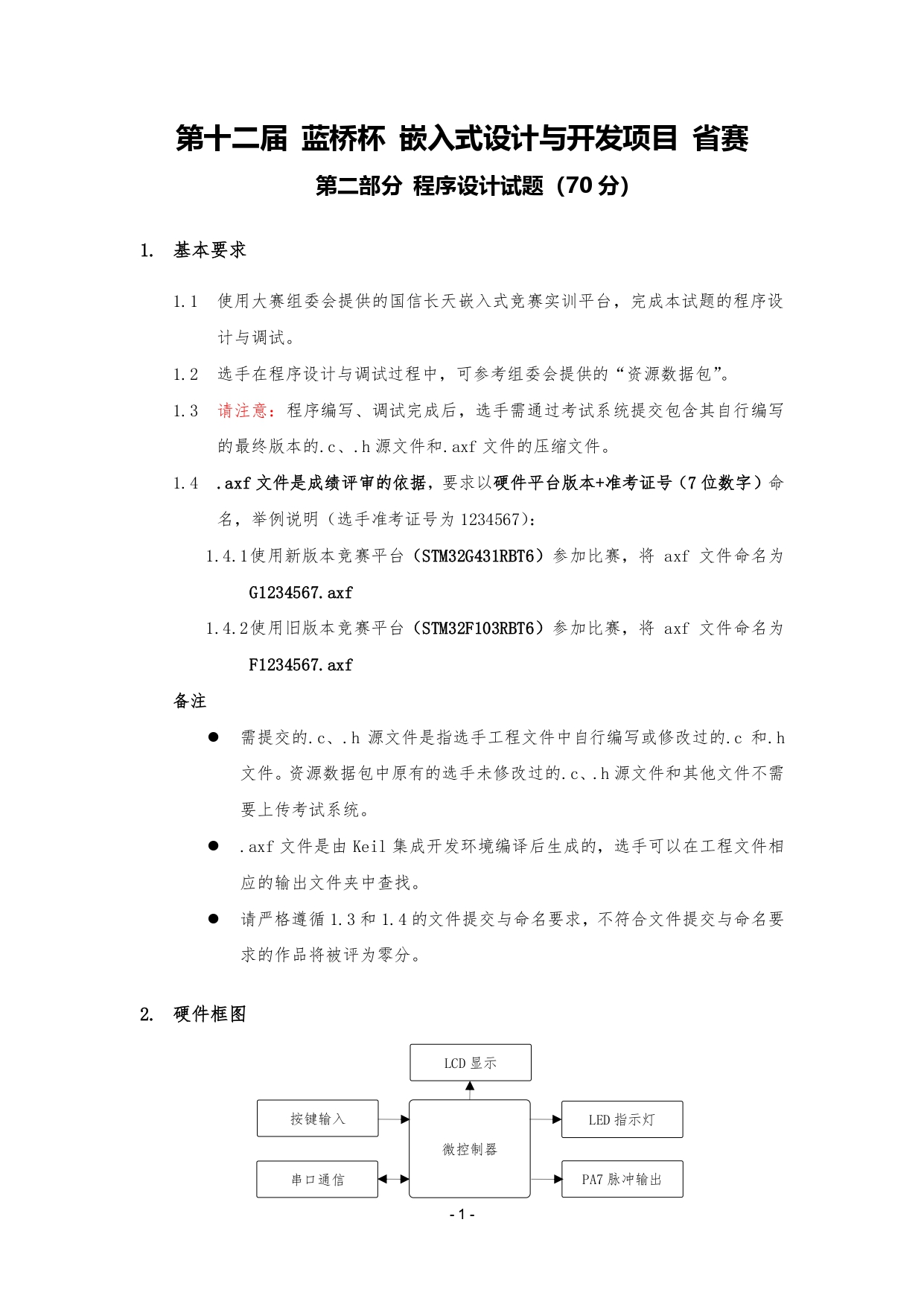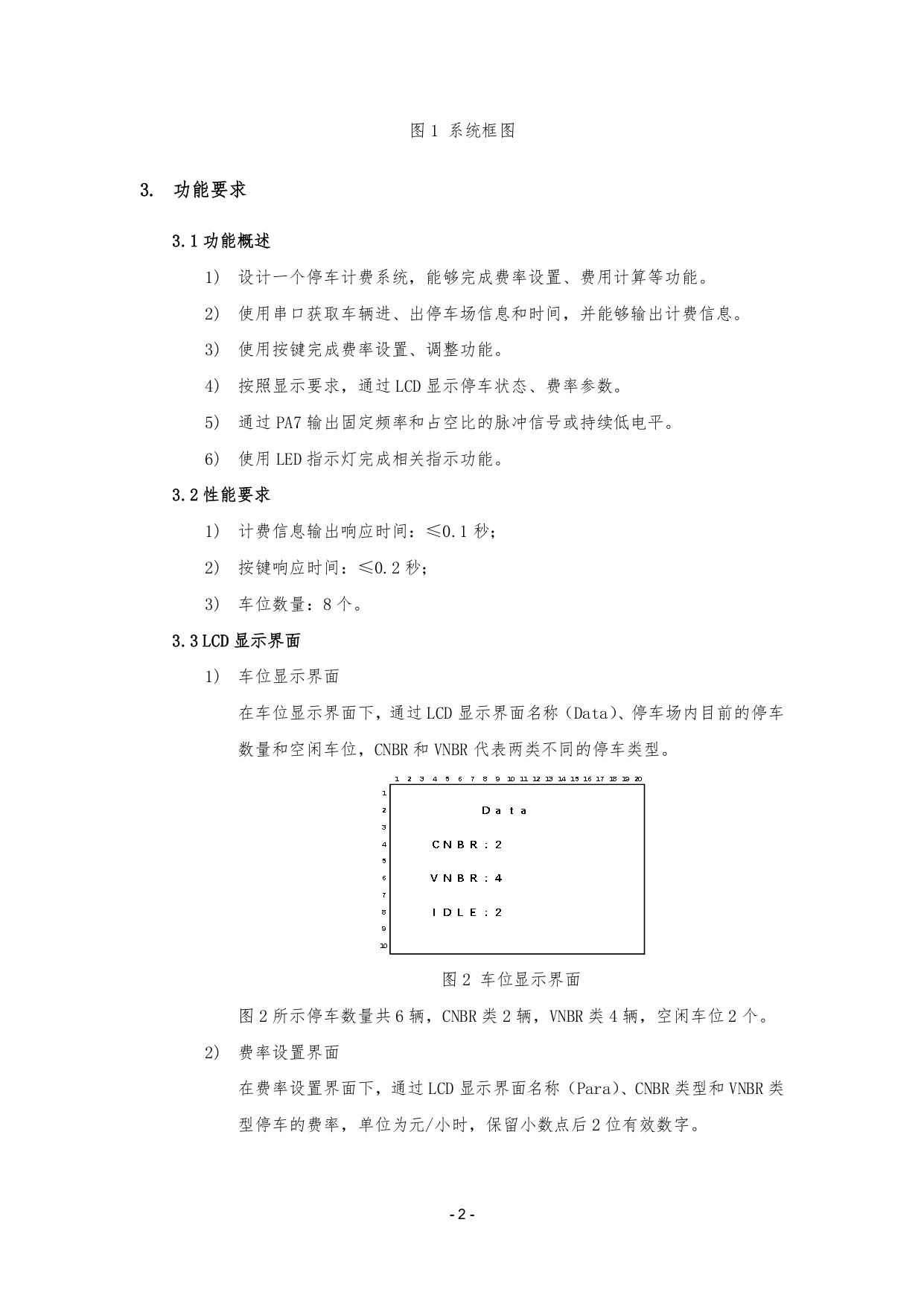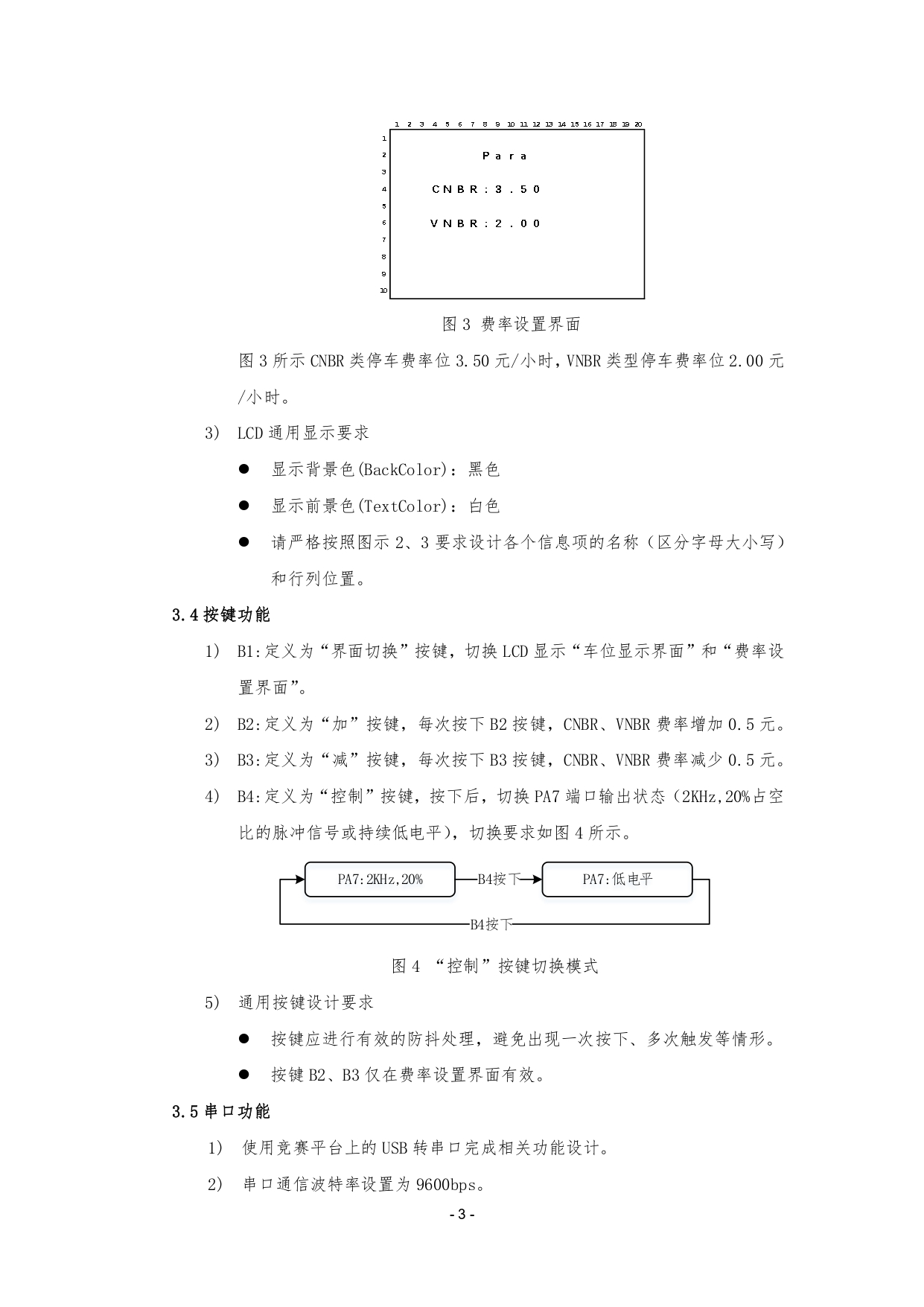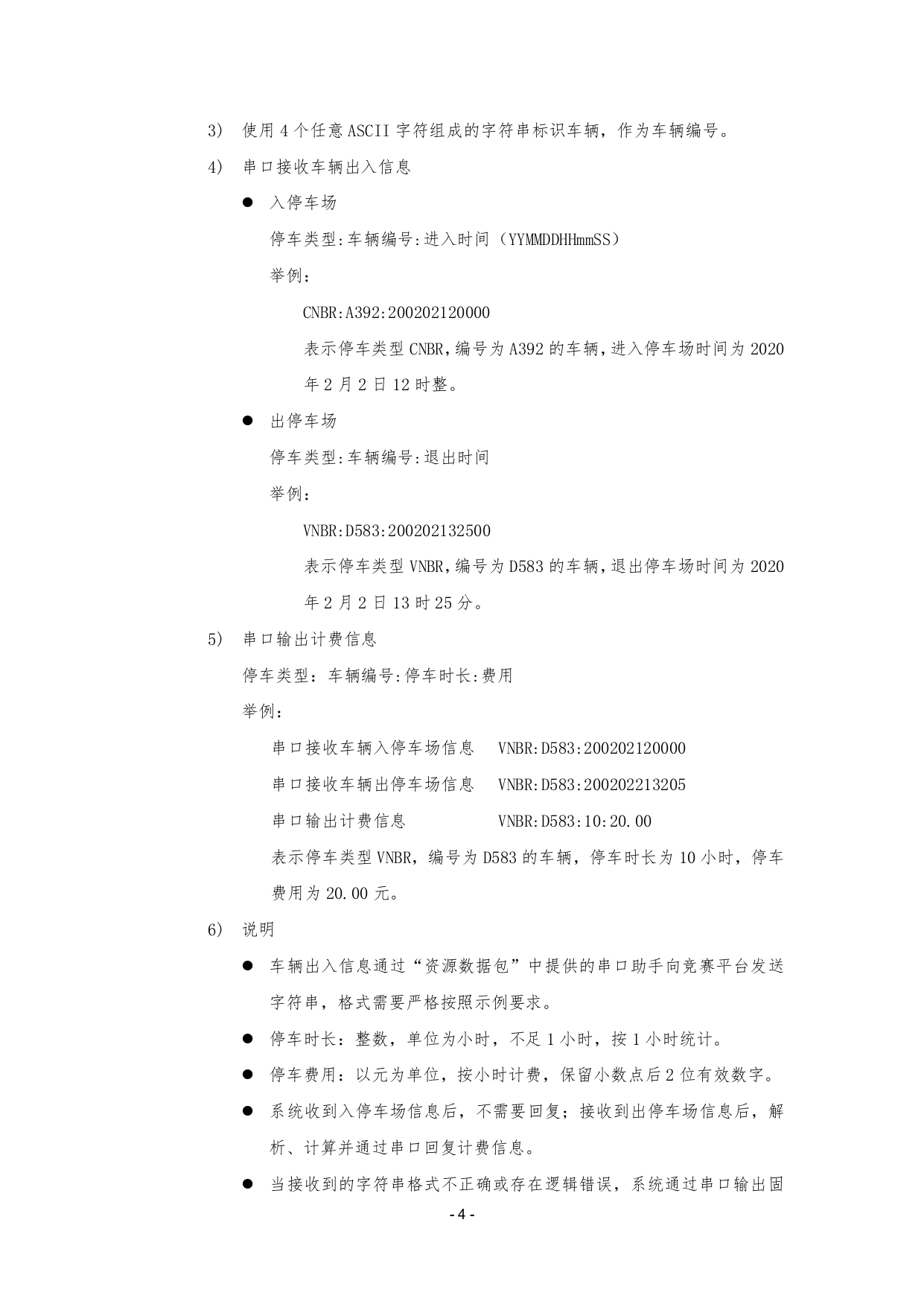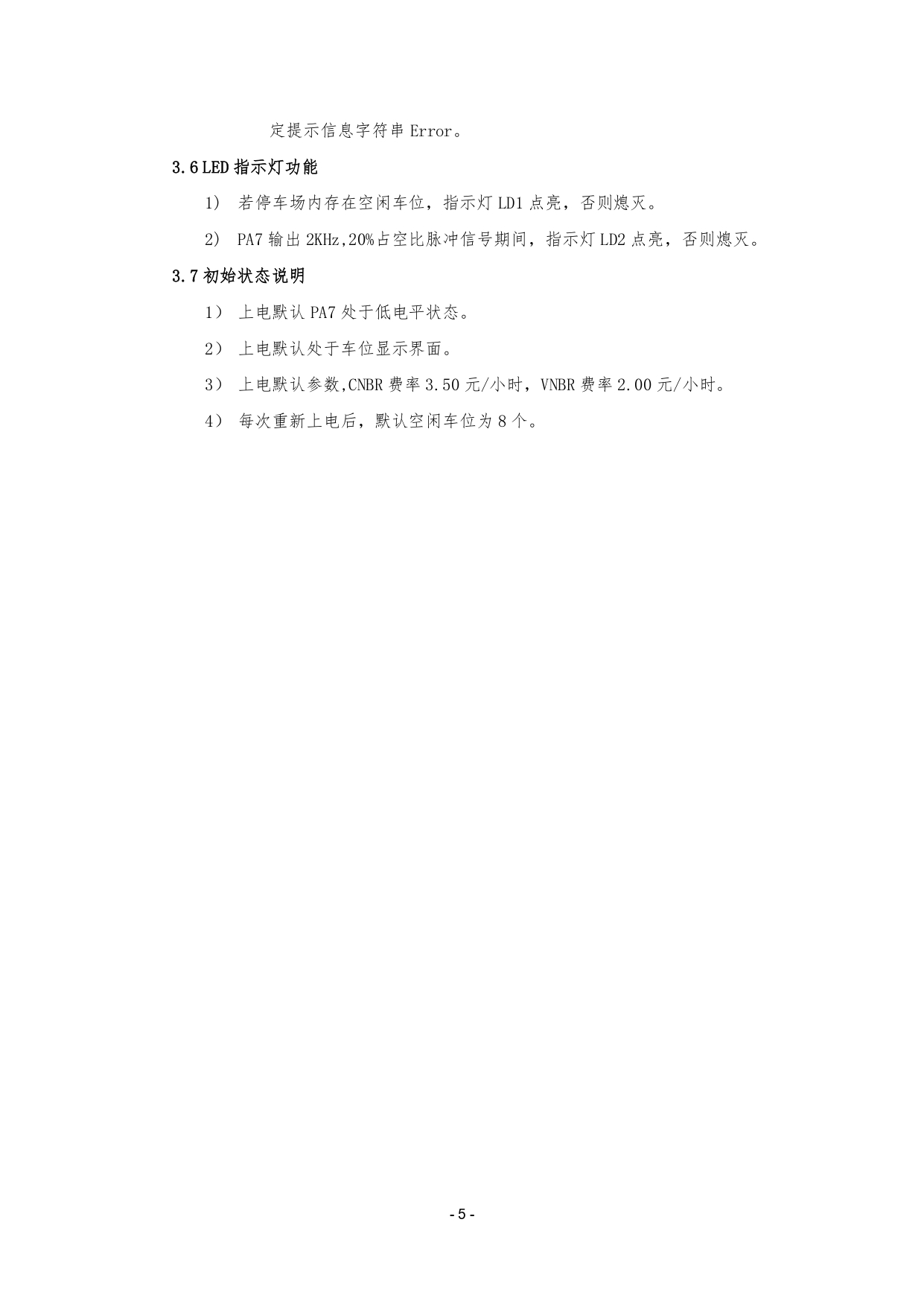二、问题总结
其他的模块应该都不会遇到什么大的问题，主要就是串口。我串口使用的是IDLE+RXNE来实现串口的不定长数据的接收的。
在实现串口的部分，首先要实现能够正确识别的进入以及出去，然后再注意一些细节的逻辑错误;

接收到正确信息的长度为22个字符，冒号的位置等等。
车型只有CNBR和VNBR两种
时间格式必须正确，并且不能出现时间的逻辑错误，如年份大于99，小时大于23等。
进入车辆信息和出去车辆信息要匹配，如进入和出去的车辆的ID相同，但是他们的车型不同是不应该的，因为对于同一个车的ID，它的信息一定全都是相同的。
出去的时间一定要晚于进入的时间。
整数，单位为小时， 不足 1 小时，按 1 小时统计。

下面附上串口部分的关键代码：
void substr(uint8_t* d_str,uint8_t* s_str,uint8_t locate,uint8_t length)
{
uint8_t i = 0;
for(i = 0; i < length; i++)
{
d_str[i] = s_str[locate + i];
}
d_str[length] = '\0';
}

uint8_t findLocate(void)	// 找一个空闲的位置
{
uint8_t i = 0;
for(i = 0; i < 8; i++)
{
if(!car[i].notEmpty)	// 如果是空闲
return i;
}
return 0xFF;
}

uint8_t isExist(uint8_t* str)		// 判断车辆是否存在
{
uint8_t i = 0;
for(i = 0; i < 8; i++)
{
if(strcmp((const char*)str,(const char*)car[i].id) == 0)
{
return i;			// 如果这辆车存在，则返回这辆车所在的车位i
}
}
return 0xFF;			// 返回0xFF表示这辆车不存在
}

_Bool checkCmd(uint8_t* str)
{
// VNBR:D583:200202120000
// 0123456789012345678901
if(RxCounter != 22)
return 0;
if((str == 'C' || str == 'V') && str == 'N' && str == 'B' && str == 'R' && str == ':' && str == ':')
{
uint8_t i;
for(i = 10; i < 22; i++)
{
if(str[i] > '9' || str[i] < '0')
return 0;
}
}
return 1;
}

void usart_proc(void)
{
if(RxFlag)
{
RxFlag = 0;

// 将接收到的字符串显示到LCD上面
//		memset(lcd_str,0,sizeof(lcd_str));
//		sprintf((char*)lcd_str,"%-20.20s",RxBuffer);
//		LCD_DisplayStringLine(Line9,lcd_str);

// VNBR:D583:200202120000
// 0123456789012345678901
if(checkCmd(RxBuffer))	// 接收到的标准信息应该是22个字符，并且只有在有空闲车位的时候有效
{
uint8_t car_id;
uint8_t car_type;
uint8_t locate = 0xFF;
uint8_t year_temp,month_temp,day_temp,hour_temp,min_temp,sec_temp;
// 将字符串中时间的信息提取出来
year_temp = (RxBuffer - '0') * 10 + (RxBuffer - '0');
month_temp = (RxBuffer - '0') * 10 + (RxBuffer - '0');
day_temp = (RxBuffer - '0') * 10 + (RxBuffer - '0');
hour_temp = (RxBuffer - '0') * 10 + (RxBuffer - '0');
min_temp = (RxBuffer - '0') * 10 + (RxBuffer - '0');
sec_temp = (RxBuffer - '0') * 10 + (RxBuffer - '0');
if(year_temp > 99 || month_temp > 12 || day_temp > 31 || hour_temp > 23 || min_temp > 59 || sec_temp > 59)
{
//				printf("shi jian ge shi error!\r\n");
goto SEND_ERROR;
}
substr(car_id,RxBuffer,5,4);		// 将车辆的编号信息提取到car_id
substr(car_type,RxBuffer,0,4);	// 将车辆的类型信息提取到car_type
locate = isExist(car_id);				// 查询这辆车是否在车库从存在

if(locate != 0xFF)	// 这辆车在车库中存在
{
int time_val;
printf("locate:%d,type:%s,id:%s\r\n",locate,car[locate].type,car[locate].id);
if(strcmp((const char *)car_type,(const char *)car[locate].type))		// 如果车辆的id和车辆的类型不同，则表示信息错误
{
//					printf("id and type pi pei error!\r\n");
goto SEND_ERROR;
}
// 假设一年365天,一个月30天，秒为单位
time_val = (year_temp - car[locate].year_in) * 365 * 24 * 3600 + (month_temp - car[locate].month_in) * 30 * 24 * 3600 + (day_temp - car[locate].day_in) * 24 * 3600 + \
(hour_temp - car[locate].hour_in) * 3600 + (min_temp - car[locate].min_in) * 60 + (sec_temp - car[locate].sec_in);
if(time_val < 0)
{
//					printf("time_val error!\r\n");
goto SEND_ERROR;
}
time_val = (time_val + 3599) / 3600;	// 换算成小时，并且不足一个小时按一个小时算

// 输出计费信息
printf("%s:%s:%d:%.2f\r\n",car[locate].type,car[locate].id,time_val,time_val / 10.0 * (RxBuffer == 'C'?CNBR_fee:VNBR_fee));

if(RxBuffer == 'C')
CNBR_cnt--;
else if(RxBuffer == 'V')
VNBR_cnt--;

memset(&car[locate],0,sizeof(car[locate]));	// 将当前结构体清空
}
else		// 这辆车在车库中不存在
{
uint8_t locate = findLocate();	// 找一个空闲的车位

if(locate == 0xFF)	// 没有找到空闲车位
{
goto SEND_ERROR;
}
//				printf("locate:%d,type:%s\r\n",locate,car[locate].type);
// 保存车辆信息
substr(car[locate].type,RxBuffer,0,4);
substr(car[locate].id,RxBuffer,5,4);
car[locate].year_in = year_temp;
car[locate].month_in = month_temp;
car[locate].day_in = day_temp;
car[locate].hour_in = hour_temp;
car[locate].min_in = min_temp;
car[locate].sec_in = sec_temp;
car[locate].notEmpty = 1;			// 标记为非空闲
if(RxBuffer == 'C')
CNBR_cnt++;
else if(RxBuffer == 'V')
VNBR_cnt++;
}
goto CMD_YES;
}
SEND_ERROR:printf("ERROR\r\n");
CMD_YES:		memset(RxBuffer,0,sizeof(RxBuffer));
RxCounter = 0;
}
}

/*	测试数据，用来测试逻辑是否有错误
1. *
VNBR:D583:200202120000
VNBR:D583:200202213205

2. *
CNBR:D593:200202120000
CNBR:D593:200203213205

3. *
VNBR:D883:200202120000
VNBR:D883:200202223205

4. *
CNBR:D588:200202120000
CNBR:D588:200202313205	会提示时间设置错误
CNBR:D588:200202223205	时间设置正确

5. *
CNBR:D580:200202120000
CNBR:D580:200202215205

6. *
VNBR:D58S:200202120000
VNBR:D58S:200203213205

7. *
CNBR:D58E:200202120000
CNBR:D58E:200204213205

8. *
CNBR:D58B:200202120000
CNBR:D58B:200205213205

9.
CNBR:D555:200202120000
CNBR:D555:200205213205
*/

三、代码（完整代码，功能全部实现，附有注释）
码云


展开全文• 2021第十二届蓝桥杯嵌入式第一场省赛赛道资源包
• 蓝桥杯单片机——第十二届蓝桥杯单片机第一场省赛 目录蓝桥杯单片机——第十二届蓝桥杯单片机第一场省赛一、赛题分析二、问题总结三、代码（完整代码，功能全部实现，附有注释） 一、赛题分析 前两天实验室的学妹把...
蓝桥杯单片机——第十二届蓝桥杯单片机第一场省赛
目录蓝桥杯单片机——第十二届蓝桥杯单片机第一场省赛一、赛题分析二、问题总结三、代码
一、赛题分析
前两天实验室的学妹把开发板还给我了，刚好今天没什么事做，就来做做今天的省赛题目。 在下是参加的去年的蓝桥杯单片机组，今年也刚参加的嵌入式组的省赛，已经好几个月没有摸单片机的板子了，以为会很生疏，看了一下之前的代码，然后做了一下今年的赛题，发现很快就找回感觉了。发现单片机组相对于嵌入式组确实要简单得多。 真的不难呀，大家一定要上手把几个基础模块过一遍，然后做两三套赛题，进决赛都挺容易的，相信我。
这一届的赛题也就中规中矩吧，已经完了前几届赛题的难度，总之这一届的还是偏简单。除了基础的数码管显示，矩阵键盘，LED之外，要是临近比赛，连这些都没有熟悉的画，自己去面壁思过吧。除此之外还考察了PCF8591的DAC功能和DS18B20。要说稍微有点难度的就是矩阵键盘和DS18B20的小数显示了吧，因为可能有些人偷懒根本就没有看过这两个部分。至于PCF8591的DAC相较于ADC简单得多，没有看过的，看一下程序应该就懂了，完整的程序可以参考第三部分。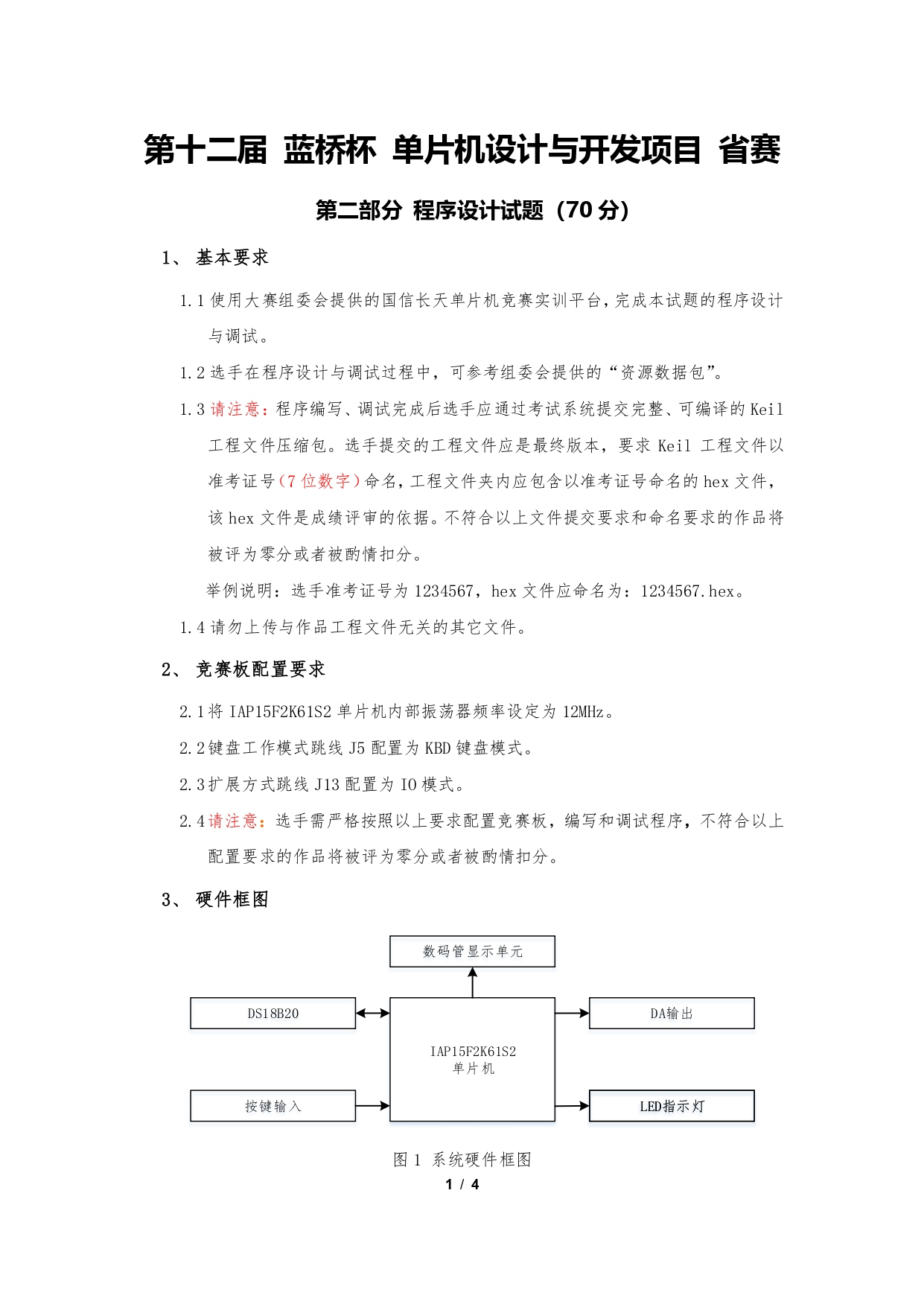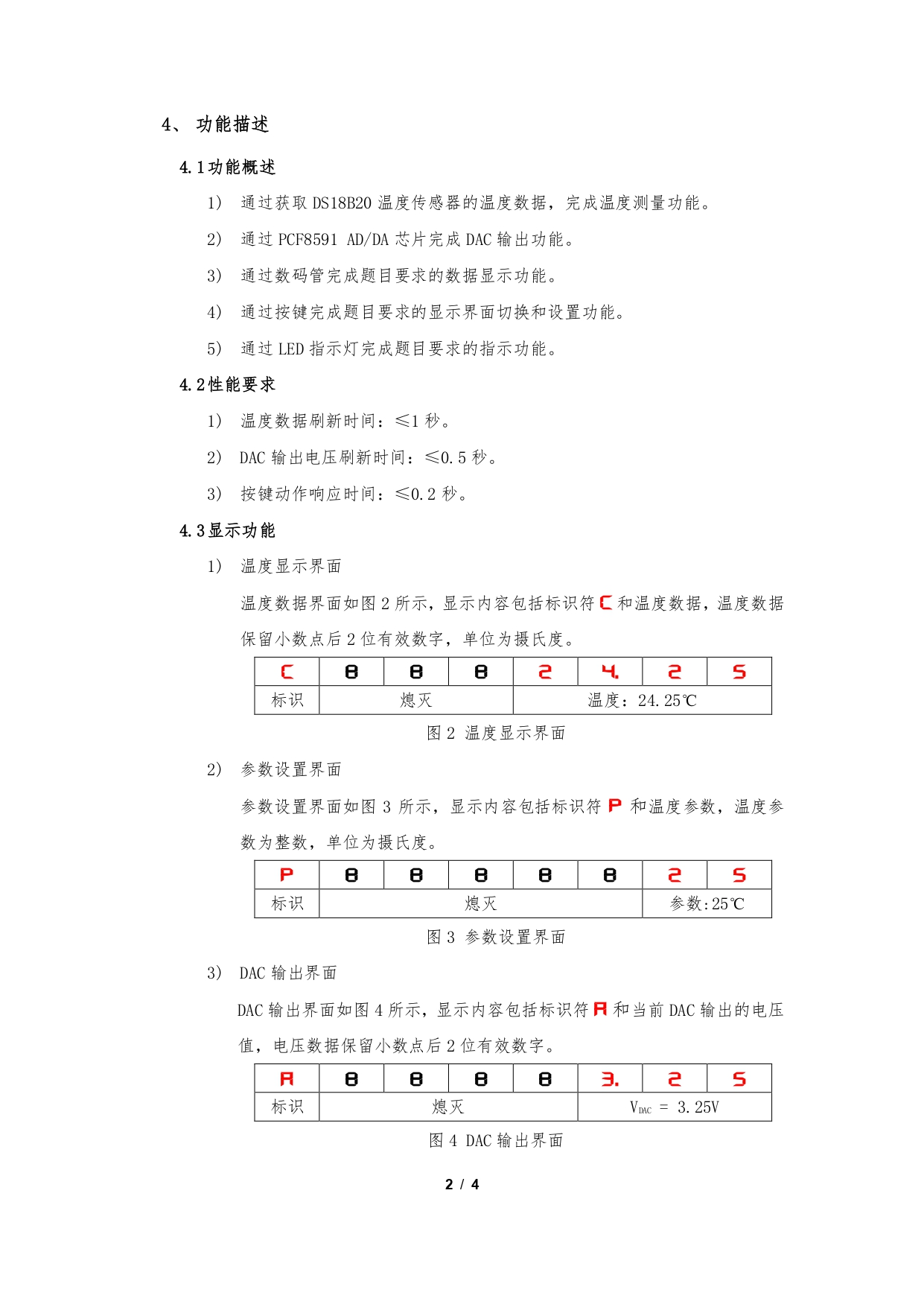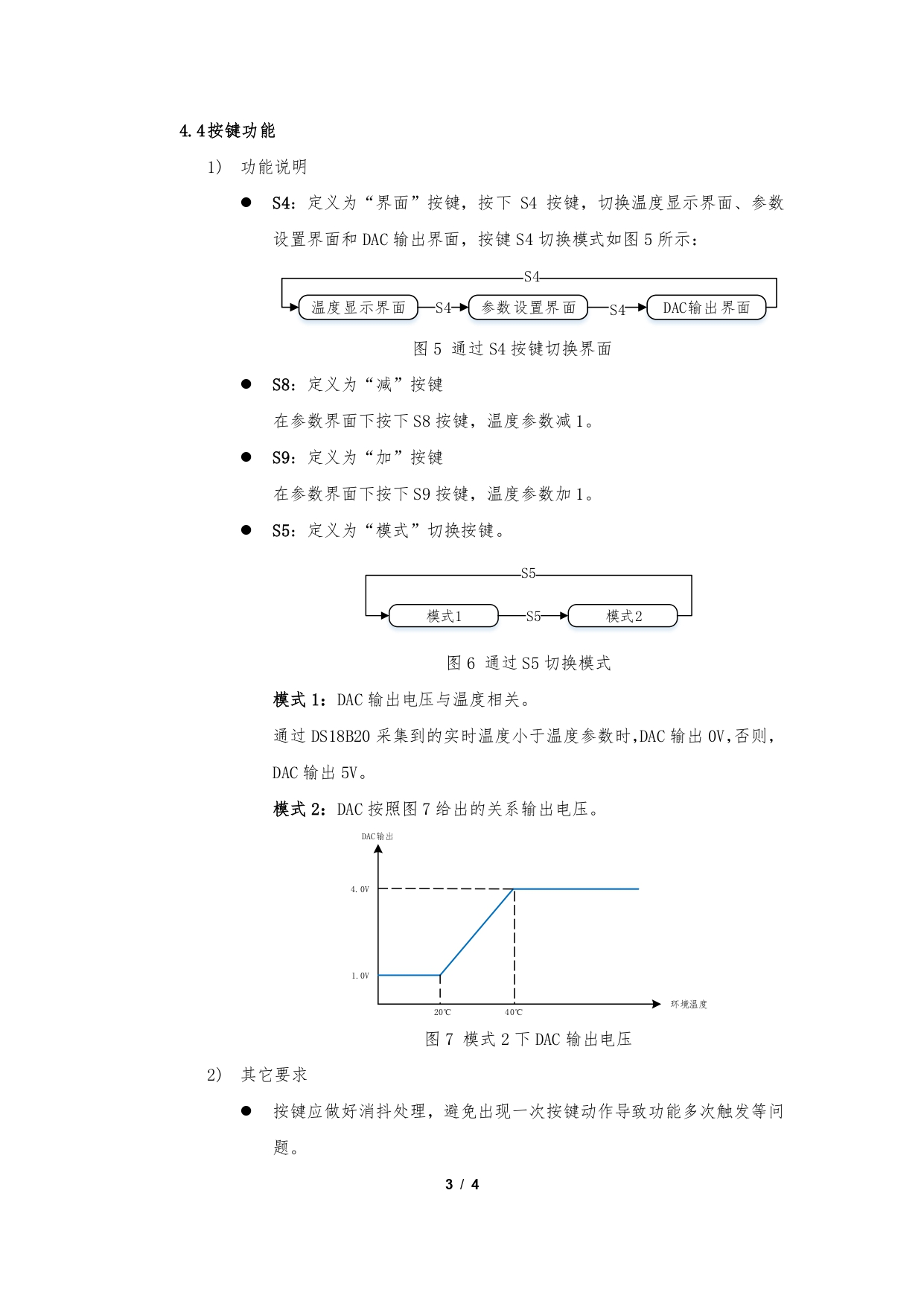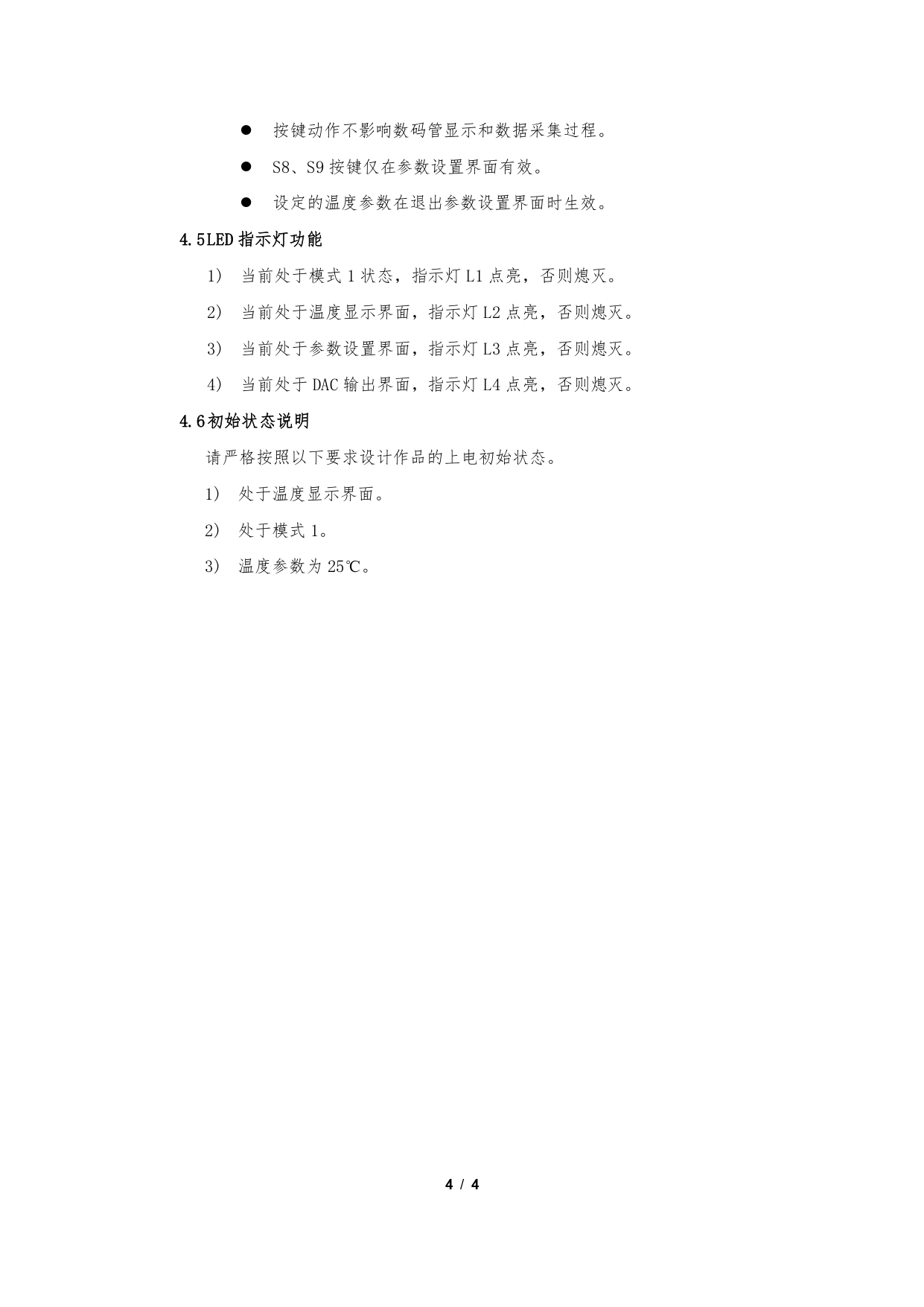二、问题总结
发现没有什么问题可以总结的，都不难…
三、代码
#include "STC15F2K60S2.h"
#include "onewire.h"
#include "iic.h"

#define u8 unsigned char
#define u16 unsigned int

#define					TEMP_INTERFACE						0
#define					DAC_INTERFACE							1
#define					SETTING_INTERFACE					2

#define get() (P3 & 0X3F) | ((P4 & 0X10) << 3) | ((P4 & 0X04) << 4)

u8 code TAB[] = {0xc0,0xf9,0xa4,0xb0,0x99,0x92,0x82,0xf8,0x80,0x90,0xbf,0xff,0xc6,0x8c,0x88};
u8 disbuf[] = {0,1,2,3,4,5,6,7,8};

u8 smg_cnt = 0;

u8 key_buf = 0;

u16 sec_tick = 0;
u8 sec_cnt = 0;

u8 interface = 0;

u8 temperature_para = 25;
u8 temperature_para_temp = 25;

bit mode = 0;

float volt = 3.25;

float temperature = 0.0;

void write_pcf8591(unsigned char dat)
{
IIC_Start();
IIC_SendByte(0x90);
IIC_WaitAck();
IIC_SendByte(0x40);
IIC_WaitAck();
IIC_SendByte(dat);
IIC_WaitAck();
IIC_Stop();
}

float read_temperature(void)
{
unsigned char high,low;
unsigned int temp;

init_ds18b20();
Write_DS18B20(0xcc);
Write_DS18B20(0x44);

init_ds18b20();
Write_DS18B20(0xcc);
Write_DS18B20(0xbe);

low = Read_DS18B20();
high = Read_DS18B20();

temp = high;
temp <<= 8;
temp |= low;

return temp * 0.0625;
}

void Delay5ms()		//@12.000MHz
{
unsigned char i, j;

i = 59;
j = 90;
do
{
while (--j);
} while (--i);
}

void set_port(u8 p2,u8 p0)
{
P2 = (P2 & 0x1F) | p2;	// 使能74HC573，与上0x1F是为了不影响其他的端口
P0 = p0;
P2 &= 0x1F;							// 将数据锁存
}

void allinit(void)
{
set_port(0x80,0xFF);	// 关闭所有LED
set_port(0xA0,0x00);	// 关闭继电器，蜂鸣器等外设
set_port(0xC0,0x00);	// 关闭数码管位选
set_port(0xE0,0x00);	// 关闭数码管段选
}

void display(void)
{
if(interface == TEMP_INTERFACE)
{
disbuf = 12;
disbuf = 11;
disbuf = 11;
disbuf = 11;
disbuf = (u16)(temperature * 100) / 1000;
disbuf = (u16)(temperature * 100) / 100 % 10;
disbuf = (u16)(temperature * 100) / 10 % 10;
disbuf = (u16)(temperature * 100) / 1000 % 10;
}
else if(interface == SETTING_INTERFACE)
{
disbuf = 13;
disbuf = 11;
disbuf = 11;
disbuf = 11;
disbuf = 11;
disbuf = 11;
disbuf = temperature_para_temp / 10;
disbuf = temperature_para_temp % 10;
}
else if(interface == DAC_INTERFACE)
{
disbuf = 14;
disbuf = 11;
disbuf = 11;
disbuf = 11;
disbuf = 11;
disbuf = (u16)(volt * 100) / 100;
disbuf = (u16)(volt * 100) / 10 % 10;
disbuf = (u16)(volt * 100) % 10;
}

}

void Timer2Init(void)		//1毫秒@12.000MHz
{
AUXR |= 0x04;		//定时器时钟1T模式
T2L = 0x20;		//设置定时初值
T2H = 0xD1;		//设置定时初值
AUXR |= 0x10;		//定时器2开始计时
}

void KBD(void)
{
u8 key_temp ;
P3 = 0X0F; P44 = 0; P42 = 0;
key_temp = get();
P3 = 0XF0; P44 = 1; P42 = 1;
key_temp |= get();

if(key_temp != 0xff && !key_buf)
{
Delay5ms();
P3 = 0X0F; P44 = 0; P42 = 0;
key_temp = get();
P3 = 0XF0; P44 = 1; P42 = 1;
key_temp |= get();
if(key_temp != 0xff && !key_buf)
{
key_buf = key_temp;
}
}
else if(key_temp == 0xff && key_buf)
{
Delay5ms();
P3 = 0X0F; P44 = 0; P42 = 0;
key_temp = get();
P3 = 0XF0; P44 = 1; P42 = 1;
key_temp |= get();
if(key_temp == 0xff && key_buf)
{
switch(key_buf)	// 这里要特别注意是key_buf，不要粗心写成了key_temp
{
//				case 0x7e: disbuf = 7; disbuf = 10; disbuf = 10; break;
//				case 0x7d: disbuf = 6; disbuf = 10; disbuf = 10; break;
case 0x7b: 				// s5
mode = !mode;

break;
case 0x77:  			// s6
switch(interface)
{
case TEMP_INTERFACE:
temperature_para_temp = temperature_para;
interface = SETTING_INTERFACE;

break;
case SETTING_INTERFACE:
temperature_para = temperature_para_temp;
interface = DAC_INTERFACE;
break;
case DAC_INTERFACE:
interface = TEMP_INTERFACE;
break;
}
break;

//				case 0xbe: disbuf = 1; disbuf = 1; disbuf = 10; break;
//				case 0xbd: disbuf = 1; disbuf = 0; disbuf = 10; break;
case 0xbb:   			// s9
temperature_para_temp++;

break;
case 0xb7:   			// s8
temperature_para_temp--;
break;

//				case 0xde: disbuf = 1; disbuf = 5; disbuf = 10; break;
//				case 0xdd: disbuf = 1; disbuf = 4; disbuf = 10; break;
//				case 0xdb: disbuf = 1; disbuf = 3; disbuf = 10; break;
//				case 0xd7: disbuf = 1; disbuf = 2; disbuf = 10; break;
//
//				case 0xee: disbuf = 1; disbuf = 9; disbuf = 10; break;
//				case 0xed: disbuf = 1; disbuf = 8; disbuf = 10; break;
//				case 0xeb: disbuf = 1; disbuf = 7; disbuf = 10; break;
//				case 0xe7: disbuf = 1; disbuf = 6; disbuf = 10; break;
}
key_buf = 0;
}
}

}

void smg_proc(void)
{
set_port(0xc0, 0x01 << smg_cnt);
if((interface == TEMP_INTERFACE && smg_cnt == 5) || (interface == DAC_INTERFACE && smg_cnt == 5))
set_port(0xe0, TAB[disbuf[smg_cnt + 1]]  & 0x7F);
else
set_port(0xe0, TAB[disbuf[smg_cnt + 1]]);
if(++smg_cnt >= 8) smg_cnt = 0;

if(++sec_tick == 1000)
{
sec_tick = 0;
(sec_cnt != 59)?(sec_cnt++):(sec_cnt = 0);
}
}

void dac_proc(void)
{
if(mode == 0)
{
if(temperature < temperature_para)
write_pcf8591(0);
else
write_pcf8591(255);
}
else
{
if(temperature < 20)
write_pcf8591(0);
else if(temperature > 40)
write_pcf8591(255);
else
write_pcf8591((6.0 / 40 * temperature - 2) / 5.0 * 255);
}
}

void led_proc(void)
{
u8 led_state = 0xff;
if(mode == 0)
led_state &= 0xFE;
if(interface == TEMP_INTERFACE)
led_state &= 0xFD;
else if(interface == SETTING_INTERFACE)
led_state &= 0xFB;
else if(interface == DAC_INTERFACE)
led_state &= 0xF7;
set_port(0x80,led_state);
}

/********************* Timer2中断函数************************/
void timer2_int (void) interrupt 12
{
smg_proc();

led_proc();
}

void main(void)
{
allinit();											// 上电初始化
Timer2Init();										// 定时器2初始化
IE2 |= 0x04;                    // 开定时器2中断
EA = 1;													// 开启总中断

while(1)
{
temperature = read_temperature();

dac_proc();

display();

KBD();
}
}

完整工程下载点我


展开全文...